# vi) Consider the following polynomials in the vector space of polynomials of degree 3 or less, P3. Pi(x) 12 +3r2 +a3 P2(x) 132 Pa(r) 1242 P4(z) = 1-r + 3r2 + 2r3 Which of the following statements...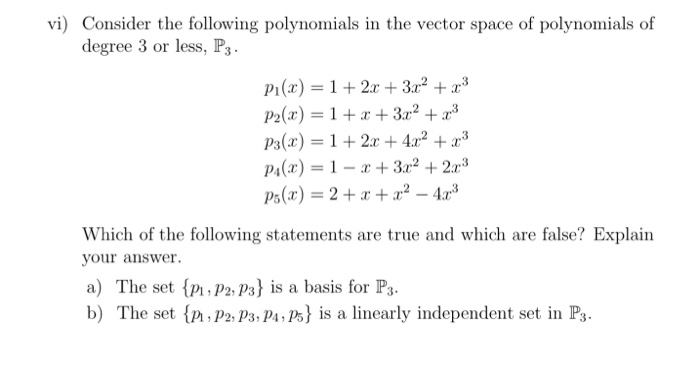vi) Consider the following polynomials in the vector space of polynomials of degree 3 or less, P3. Pi(x) 12 +3r2 +a3 P2(x) 132 Pa(r) 1242 P4(z) = 1-r + 3r2 + 2r3 Which of the following statements are true and which are false? Explain your answer. a) The set {Pi, P2,P3} is a basis for P3. b) The set {Pi,P2, p3,P4,P5} İs a linearly independent set in P3.

Given that: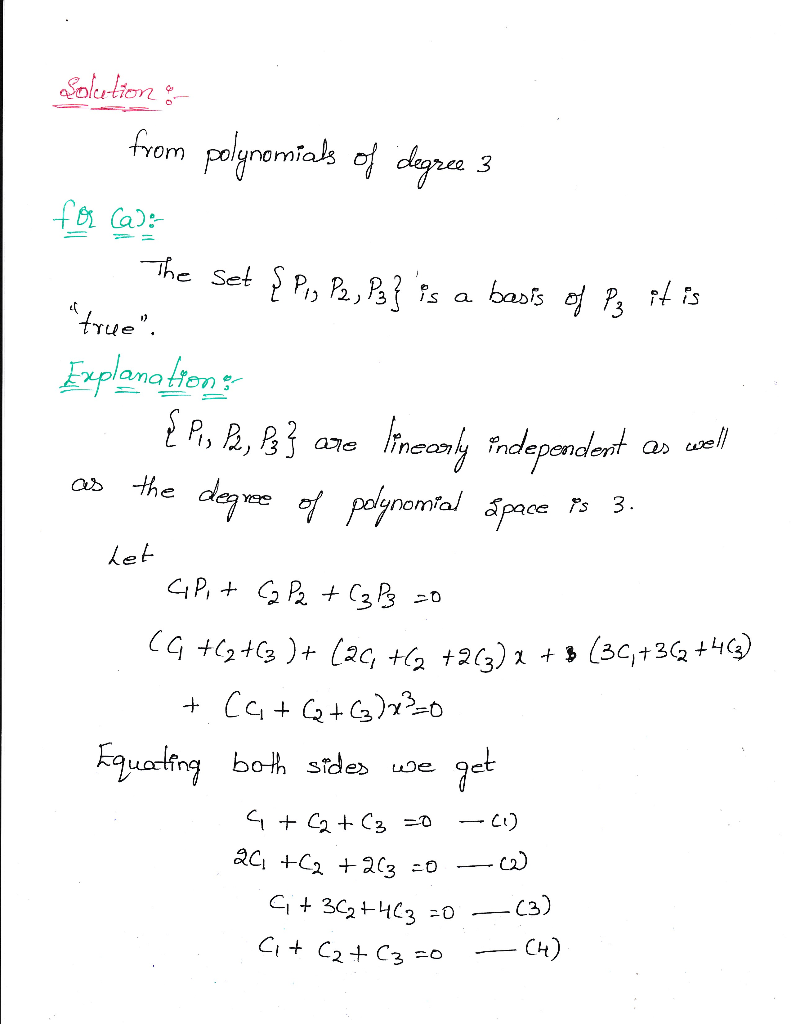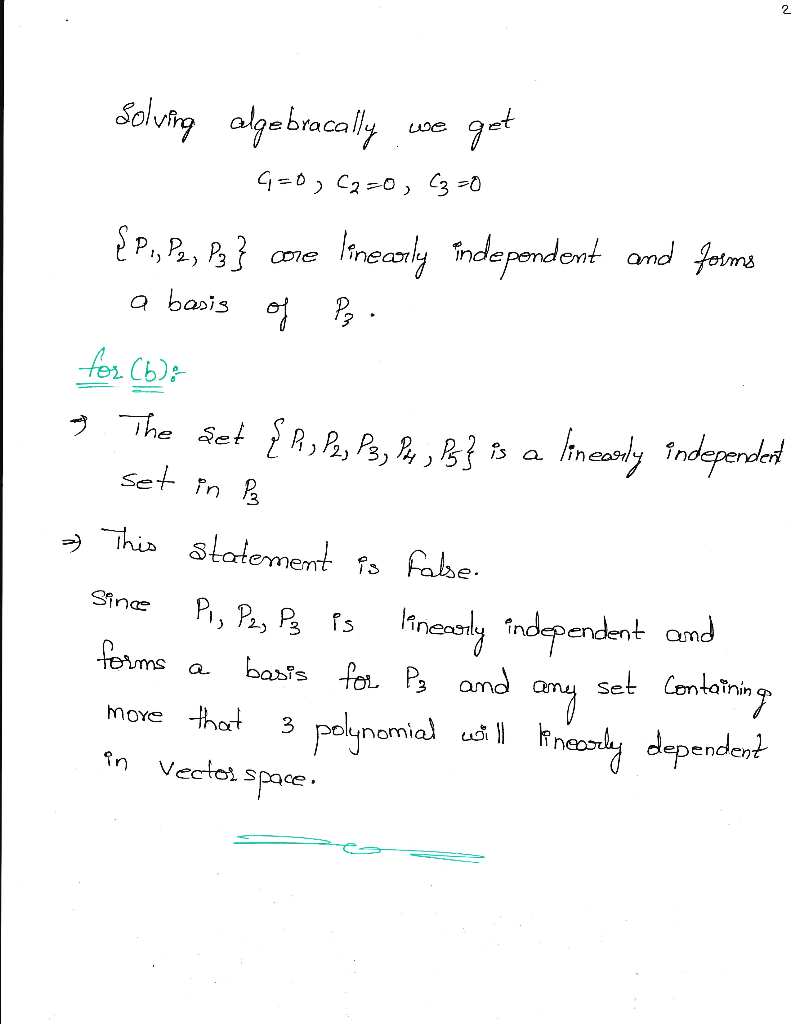##### Add Answer of: vi) Consider the following polynomials in the vector space of polynomials of degree 3 or less, P3. Pi(x) 12 +3r2 +a3 P2(x) 132 Pa(r) 1242 P4(z) = 1-r + 3r2 + 2r3 Which of the following statements...
Similar Homework Help Questions
• ### let P3 denote the vector space of polynomials of degree 3 or less, with an inner product defined by 14. Let Ps denote the vector space of polynomials of degree 3 or less, with an inner product def...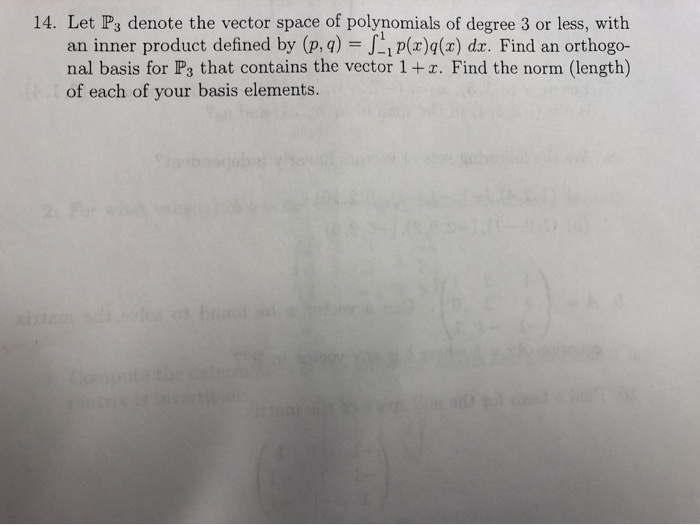let P3 denote the vector space of polynomials of degree 3 or less, with an inner product defined by 14. Let Ps denote the vector space of polynomials of degree 3 or less, with an inner product defined by (p, q) Ji p(x)q(x) dr. Find an orthogo- nal basis for Ps that contains the vector 1+r. Find the norm (length) of each of your basis elements 14. Let Ps denote the vector space of polynomials of degree 3 or less,...

• ### Let V be the vector space of the polynomials of K [t] of degree less than 3, that is, the form p (x) = a2t2 + a1t1 + a0t0. Investigate the linear independence of the polynomials: p1 (t) = 1t2 + 0t1 +...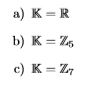Let V be the vector space of the polynomials of K [t] of degree less than 3, that is, the form p (x) = a2t2 + a1t1 + a0t0. Investigate the linear independence of the polynomials: p1 (t) = 1t2 + 0t1 + 1t0, p2 (t) = 2t2 + 2t1 + 0t0, p3 (t) = 4t2 + 1t1 + 3t0 for: *b) The modulo operation on 5 *c) The modulo operation on 7 a) K-R b) K c) K a)...

• ### 7. Let V = Pa(R), the vector space of polynomials over R of degree less than 2, with inner product Define φ E p by φ(g)-g(-1) a) By direct calculation, find f e V such that (S)-dg). You are given...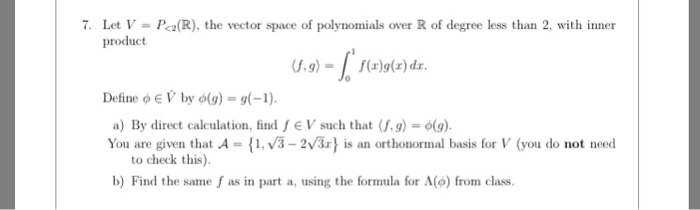7. Let V = Pa(R), the vector space of polynomials over R of degree less than 2, with inner product Define φ E p by φ(g)-g(-1) a) By direct calculation, find f e V such that (S)-dg). You are given that A 1, V3-2v) is an orthonormal basis for V (you do not need to check this). b) Find the same f as in part a, using the formula for A(6) from class. 7. Let V = Pa(R), the vector...

• ### Please provide answer in neat handwriting. Thank you Let P2 be the vector space of all polynomials with degree at most 2, and B be the basis {1,T,T*). T(p(x))-p(kr); thus, Consider the linear operato...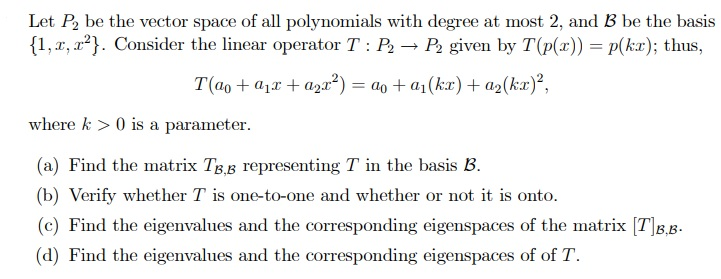Please provide answer in neat handwriting. Thank you Let P2 be the vector space of all polynomials with degree at most 2, and B be the basis {1,T,T*). T(p(x))-p(kr); thus, Consider the linear operator T : P) → given by where k 0 is a parameter (a) Find the matrix Tg,b representing T in the basis B (b) Verify whether T is one-to-one and whether or not it is onto. (c) Find the eigenvalues and the corresponding eigenspaces of the...

• ### Problem 3. Let D be the vector space of all differentiable function R wth the usual pointwise add...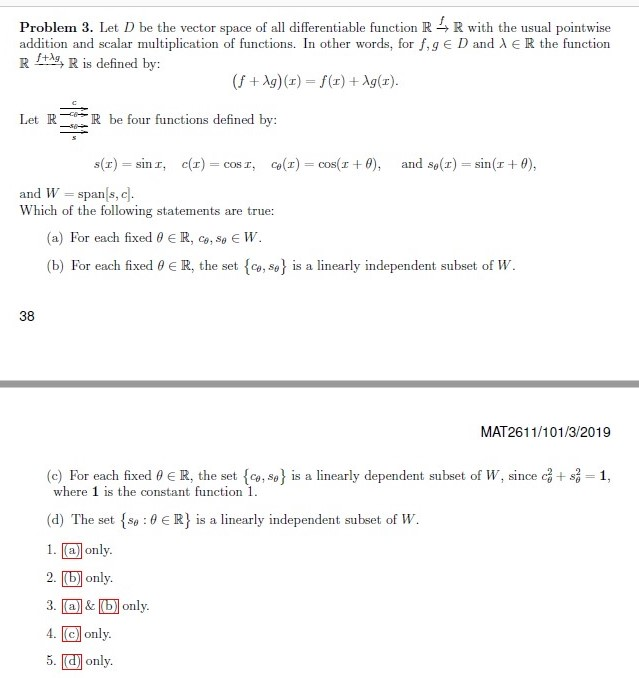Problem 3. Let D be the vector space of all differentiable function R wth the usual pointwise addition and scalar multiplication of functions. In other words, for f, g E D and λ E R the function R defined by: (f +Ag) ()-f(r) +Ag(x) Let R be four functions defined by: s(x)-: sin 11 c(r) : cosz, co(z)--cos(z + θ), and so(r) sin(z + θ), and Wspanls, c Which of the following statements are true: (a) For each fixed θ...

• ### Prove the following: (a) Let V be a vector space of dimension 3 and let {v,U2,U3} be a basis for V. Show that u2, u2 -2+s and uvi also form a basis for V (b) Show that1-,1-2,1-- 2 is a basis for P2[r...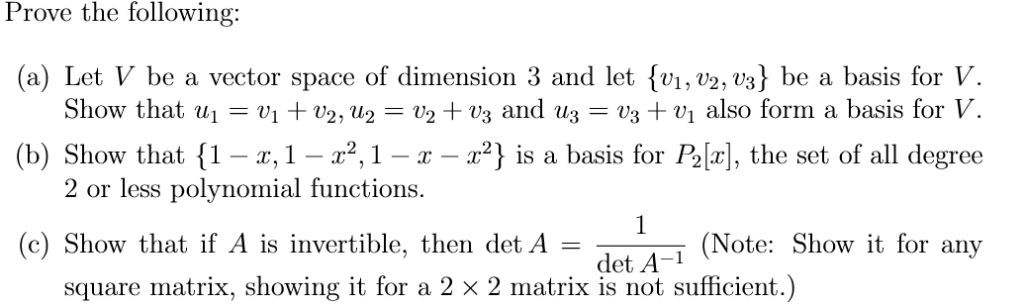Prove the following: (a) Let V be a vector space of dimension 3 and let {v,U2,U3} be a basis for V. Show that u2, u2 -2+s and uvi also form a basis for V (b) Show that1-,1-2,1-- 2 is a basis for P2[r], the set of all degree 2 or less polynomial functions. (c) Show that if A is invertible, then det A (Note: Show it for any det A-1 square matrix, showing it for a 2 x 2 matrix...

Need Online Homework Help?